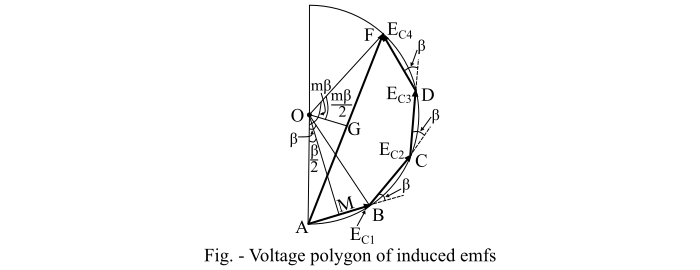# Distribution Factor or Breadth Factor ŌĆō Definition, Formula and Example

In a concentrated winding, the coil sides of a given phase are concentrated in a single slot under a given pole. The induced EMFs in individual coils are in phase with each other. Therefore, these EMFs may be added arithmetically. To determine the induced EMF per phase in case of concentrated winding, a given coil EMF is multiplied by the number of series connected coils per phase.

In actual practice, the coils of the windings of the alternator in each phase are not concentrated in a single slot, but are distributed in a number of slots in space to form a polar group under each pole. The EMFs induced in coil sides constituting a polar group, which are not in phase but differ by an angle equal to the angular displacement (β) of the slots. The total voltage generated in any phase will be the phasor sum of the individual coil voltages.

The distribution factor or breadth factor is defined as the ratio of the EMF induced with the distributed winding to the EMF induced with the concentrated winding, i.e.,

$$\mathrm{Distribution\:factor, ­Øæś_{­Øææ} =\frac{EMF\: with\:distributed\:winding}{EMF\:with\:concentrated \:winding}… (1)}$$

Let,

$$\mathrm{­ØæÜ = Slots\:per\:pole\:per\:phase =\frac{slots}{pole \times phase}}$$

$$\mathrm{β = Angular\:displacement\:between \:adjacent\:slots\:in\:electrical\:degrees}$$

$$\mathrm{β =\frac{180° \times poles}{slots}}$$

Hence, one phase of the winding consists of coils arranged in m consecutive slots. The EMFs ­ØÉĖ­ØÉČ1, ­ØÉĖ­ØÉČ2, ­ØÉĖ­ØÉČ3,… are the individual coil EMFs. Each coil EMF ­ØÉĖ­ØÉČ will be out of phase with the next coil EMF by the slot pitch (β).Refer the voltage polygon of the induced EMFs in four coils of a group that is shown in the figure. Here, the EMFs ­ØÉĖ­ØÉČ1, ­ØÉĖ­ØÉČ2, ­ØÉĖ­ØÉČ3 and ­ØÉĖ­ØÉČ4 are represented by the phasors AB, BC, CD and DF respectively. Each of these phasors is a chord of a circle with centre O and subtends an angle β at the centre O. The phasor AF represents the phasor sum that gives the resultant winding voltage and subtends an angle mβ at O.

Now, the distribution factor can also be given as

$$\mathrm{­Øæś_{­Øææ} =\frac{Phasor\:sum\:of\:coil \:voltages\:per\:phase}{Arithmetic\:sum\:of\:coil \:voltages\:per\:phase}… (2)}$$

The arithmetic sum of individual coil voltages is given by,

$$\mathrm{Arithmetic\:sum\:of\:coil\:voltages = ­ØæÜ­ØÉĖ_{­ØÉČ} = ­ØæÜ ŌłÖ ­ØÉ┤­ØÉĄ = ­ØæÜ ŌłÖ (2­ØÉ┤­ØæĆ)}$$

$$\mathrm{\Rightarrow\:Arithmetic\:sum\:of\:coil \:voltages = 2­ØæÜ ŌłÖ ­Øæé­ØÉ┤\:sin \angle ­ØÉ┤­Øæé­ØæĆ}$$

$$\mathrm{= 2­ØæÜ ŌłÖ ­Øæé­ØÉ┤\:sin(β/2)}$$

And the phasor sum of individual coil voltages is given by,

$$\mathrm{Phasor\:sum\:of\:coil\:voltages = ­ØÉ┤­ØÉ╣ = 2­ØÉ┤­ØÉ║ = 2 ŌłÖ ­Øæé­ØÉ┤\:sin \angle ­ØÉ┤­Øæé­ØÉ║}$$

$$\mathrm{= 2 ŌłÖ ­Øæé­ØÉ┤\:sin(­ØæÜ β/2)}$$

Therefore, the distribution factor can be written as,

$$\mathrm{­Øæś_{­Øææ} =\frac{Phasor\:sum\:of \:coil \:voltages \:per\: phase}{Arithmetic \:sum \:of\: coil \:voltages \:per \:phase}=\frac{2 ŌłÖ ­Øæé­ØÉ┤ sin(­ØæÜ β/2)}{2­ØæÜ ŌłÖ ­Øæé­ØÉ┤\:sin(β/2)}}$$

$$\mathrm{\Rightarrow\:­Øæś_{­Øææ} =\frac{sin(­ØæÜ β/2)}{m\:sin(β/2)}… (3)}$$

From Eq. (3), it can be noted that the distribution factor for a given number of phases is dependent only on the number of distributed slots under a given pole. It is independent of the type of winding, number of turns per coil etc. With the increase in the number of slots per pole, the distribution factor decreases.

## Numerical Example

A 3-phase, 50 Hz, 6-pole alternator has a star connected winding with 180 slots and 10 conductors per slot. Assume that the coils are full pitch coil. Determine the winding distribution factor.

Solution

The slots per pole per phase are given by,

$$\mathrm{­ØæÜ =\frac{slots}{pole \times phase}=\frac{180}{6 \times 3}= 10}$$

And the slot pitch is,

$$\mathrm{β =\frac{180° \times poles}{slots}=\frac{180° \times 6}{180}= 6°}$$

Therefore, the winding distribution factor is given by,

$$\mathrm{­Øæś_{­Øææ} =\frac{sin(­ØæÜ β/2)}{m\:sin(β/2)}=\frac{sin(\frac{10 × 6}{2})}{10 × sin(\frac{6°}{2})}=\frac{0.5}{0.523}= 0.956}$$